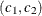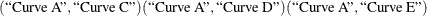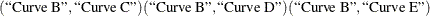#### Grouped-Name-Lists

A grouped-name-list specifies multiple scenarios for names in two or more groups. The list can assume one of two general forms, a crossed version and a matched version.

##### Crossed Grouped-Name-Lists

The crossed version of a grouped name list consists of a series of name-lists (see the section Name-Lists), one representing each group, with groups separated by a vertical bar (|). The values for each group represent multiple scenarios for that group, and the scenarios for each individual group are crossed to produce the set of all scenarios for the analysis option. For example, you can specify the following six scenarios for the survival curvesof two groupsas follows:

```   GROUPSURVIVAL = "Curve A" "Curve B" | "Curve C" "Curve D"
"Curve E"
```
##### Matched Grouped-Name-Lists

The matched version of a grouped name list consists of a series of name lists, each enclosed in parentheses. Each list consists of a name for each group and represents a single scenario for the analysis option. Multiple scenarios for the analysis option are represented by multiple lists. For example, you can express the crossed grouped-name-list

```   GROUPSURVIVAL = "Curve A" "Curve B" | "Curve C" "Curve D"
"Curve E"
```

alternatively in a matched format:

```   GROUPSURVIVAL = ("Curve A" "Curve C")
("Curve A" "Curve D")
("Curve A" "Curve E")
("Curve B" "Curve C")
("Curve B" "Curve D")
("Curve B" "Curve E")
```

The matched version is particularly useful when you want to include only a subset of all combinations of individual group values. For example, you might want to pair Curve A only with Curve C, and Curve B only with Curve D and Curve E:

```   GROUPSURVIVAL = ("Curve A" "Curve C")
("Curve B" "Curve D")
("Curve B" "Curve E")
```